NEET  >  NEET Previous Year Questions (2014-21): Kinetic Theory

# NEET Previous Year Questions (2014-21): Kinetic Theory - Notes | Study Physics Class 11 - NEET

 1 Crore+ students have signed up on EduRev. Have you?

Q.1. Match column - I and column - II and choose the correct match from the given choices.A: (A) - (Q), (B) - (P), (C) - (S), (D) - (R)
B: (A) - (R), (B) - (Q), (C) - (P), (D) - (S)
C: (A) - (R), (B) - (P), (C) - (S), (D) - (Q)
D: (A) - (Q), (B) - (R), (C) - (S), (D) - (P)
Ans:
A
Root mean square speed of gas moleculesQ.2. A cylinder contains hydrogen gas at pressure of 249 kPa and temperature 27°C.
Its density is : (R = 8.3 J mol-1 K-1)     
A: 0.1 kg/m3

B: 0.02 kg/m3
C: 0.5 kg/m3
D: 0.2 kg/m3
Ans: D
∴ PV = nRT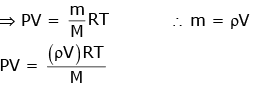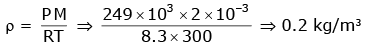Q.3. The mean free path for a gas, with molecular diameter d and number density n can be expressed as :     
A: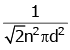B: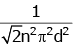C: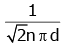D: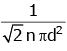Ans:
D
The mean free path for gas is given by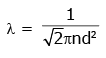Q.4. Increase in temperature of a gas filled in a container would lead to:    
A: Increase in its mass
B: Increase in its kinetic energy
C: Decrease in its pressure
D: Decrease in intermolecular distance
Ans:
B
Solution: Increase in temperature would lead to the increase in kinetic energy of gas (assuming far as to be ideal) as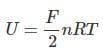Q.5. At what temperature will the rms speed of oxygen molecules become just sufficient for escaping from the Earth's atmosphere ? (Given: Mass of oxygen molecule (m) = 2.76 × 10–26 kg Boltzmann's constant kB = 1.38 × 10–23 J K–1):-    
A: 2.508 × 104 K
B: 8.360 × 104 K
C: 5.016 × 104 K
D: 1.254 × 104 K
Ans:
B
Solution: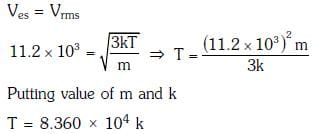Q.6. The de-Broglie wavelength of a neutron in thermal equilibrium with heavy water at a temperature T(Kelvin) and mass m, is:-    
A: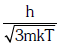B: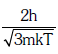C: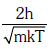D: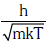Ans:
A
Solution: Kinetic energy of thermal neutron with equilibrium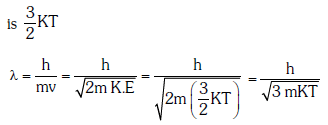Q.7. A gas mixture consists of 2 moles of O2 and 4 moles of Ar at temperature T. Neglecting all vibrational modes, the total internal energy of the system is:-    
A: 15 RT
B: 9 RT
C: 11 RT
D: 4 RT
Ans:
C
Solution:Q.8. The molecules of a given mass of a gas have r.m.s. velocity of 200 ms-1 at 27°C and 1.0 x 10-5 Nm-2 pressure. When the temperature and pressure of the gas are respectively, 127°C and 0.05 x 105Nm2, the r.m.s. velocity of velocity of its molecules in ms-1 is;    
A: 100 / 3
B: 100 √2
C: 400 / √3
D: 100√2 / 3
Ans:
C
Solution:
It is given that,
vrms = 200 m/s
T1 = 300 K
P1 = 105 N/m2
rms velocity of gas molecules,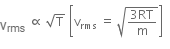For two different cases,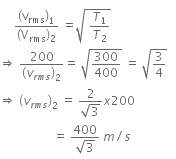It is given that,
vrms = 200 m/s
T1 = 300 K
P1 = 105 N/m2
rms velocity of gas molecules,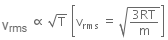For two different cases,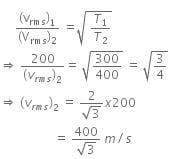Q.9. The ratio of the specific heats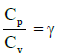in terms of degrees of freedom (n) is given by:    
A: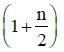B: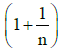C: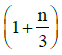D: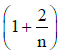Ans:
D
Solution: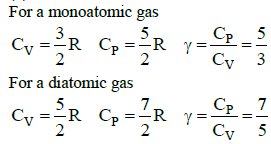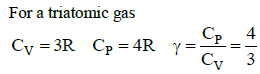This fits into the pattern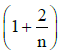, where n is the number of the degrees of freedom

Q.10. The mean free path of molecules of a gas,(radius ‘r’) is inversely proportional to:    
A: r
B: √r
C: r3
D: r2
Ans: D

Solution: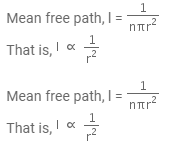The document NEET Previous Year Questions (2014-21): Kinetic Theory - Notes | Study Physics Class 11 - NEET is a part of the NEET Course Physics Class 11.
All you need of NEET at this link: NEET

## Physics Class 11

127 videos|464 docs|210 tests
 Use Code STAYHOME200 and get INR 200 additional OFF

## Physics Class 11

127 videos|464 docs|210 tests

### How to Prepare for NEET

Read our guide to prepare for NEET which is created by Toppers & the best Teachers

Track your progress, build streaks, highlight & save important lessons and more!

,

,

,

,

,

,

,

,

,

,

,

,

,

,

,

,

,

,

,

,

,

;